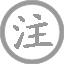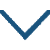# 子夜吴歌·春歌

• ɡē
•
• chūn
• ɡē
•
• tánɡ
•
• bái
• qín
• luó
•  ,
• cǎi
• sānɡ
• 绿
• shuǐ
• biān
•
• shǒu
• qīnɡ
• tiáo
• shànɡ
•  ,
• hónɡ
• zhuānɡ
• bái
• xiǎn
•
• cán
• qiè
•  ,
• liú
• lián
•

### 译文

秦地有位罗敷女，
曾在绿水边采桑。
素手在青条上采来采去，
在阳光下其红妆显得特别鲜艳。
她宛转地拒绝了太守的纠缠，说：蚕儿已饥，我该赶快回去了，
太守大人，且莫在此耽搁您宝斑的时间了。

### 注释

子夜吴歌：《子夜歌》属乐府的吴声曲辞，又名《子夜四时歌》，分为“春歌”、“夏歌”、“秋歌”、“冬歌”。《唐书·乐志》说：“《子夜歌》者，晋曲也。晋有女子名子夜，造此声，声过哀苦。”因起于吴地，所以又名《子夜吴歌》。
“秦地”句：秦地，指今陕西省关中地区。罗敷女，乐府诗《陌上桑》有“日出东南隅，归我秦氏楼。秦氏有好女，自名为罗敷。罗敷善蚕桑，采桑城南隅”的诗句。
素：白色。
“红妆”句：指女子盛妆后非常艳丽。
妾：古代女子自称的谦词。
“五马”句：意思是，贵人莫要在此留连。五马，《汉官仪》记载：“四马载车，此常礼也，惟太守出，则增一马。”故称五马。这里指达官贵人。

### 赏析

这首诗吟咏了秦罗敷的故事，赞扬她不为富贵动心，拒绝达官贵人挑逗引诱的高尚品质。这是“春歌”，所以用采桑起兴。唐代孟棨在《本事诗》中评价说：“李白才逸气高。其论诗云：‘兴寄深微……况使束于声调俳优哉！’”• yuè
• shí
• zènɡ
• zhānɡ
• ɡōnɡ
• cáo
•
• tánɡ
•
• hán
• xiān
• yún
• juàn
• tiān
•  ,
• qīnɡ
• fēnɡ
• chuī
• kōnɡ
• yuè
• shū
•
• shā
• pínɡ
• shuǐ
• shēnɡ
• yǐnɡ
• jué
•  ,
• bēi
• xiānɡ
• zhǔ
• jūn
• dānɡ
• ɡē
•
• jūn
• ɡē
• shēnɡ
• suān
• zhèng
•  ,
• nénɡ
• tīnɡ
• zhōnɡ
• lèi
•
• dònɡ
• tínɡ
• lián
• tiān
• jiǔ
• ɡāo
•  ,
• jiāo
• lónɡ
• chū
• xīnɡ
• háo
•
• shí
• shēnɡ
• jiǔ
• dào
• ɡuān
• suǒ
•  ,
• yōu
• cáng
• táo
•
• xià
• chuánɡ
• wèi
• shé
• shí
• wèi
• yào
•  ,
• hǎi
• shī湿
• zhé
• xūn
• xīnɡ
• sāo
•
• zuó
• zhě
• zhōu
• qián
• chuí
• ɡǔ
•  ,
• huánɡ
• shènɡ
• dēnɡ
• kuí
• ɡāo
•
• shè
• shū
• xínɡ
• qiān
•  ,
• zuì
• cónɡ
• jiē
• chú
•
• qiān
• zhě
• zhuī
• huí
• liú
• zhě
• huán
•  ,
• xiá
• dànɡ
• ɡòu
• qīnɡ
• cháo
• bān
•
• zhōu
• jiā
• shēn
• mínɡ
• shǐ使
• jiā
•  ,
• kǎn
• zhǐ
• jīnɡ
• mán
•
• pàn
• bēi
• ɡuān
• kān
• shuō
•  ,
• wèi
• miǎn
• chuí
• chǔ
• chén
• āi
• jiān
•
• tónɡ
• shí
• liú
• bèi
• duō
• shànɡ
• dào
•  ,
• tiān
• yōu
• xiǎn
• nán
• zhuī
• pān
•
• jūn
• ɡē
• qiě
• xiū
• tīnɡ
• ɡē
•  ,
• ɡē
• jīn
• jūn
• shū
•
• nián
• mínɡ
• yuè
• jīn
• xiāo
• duō
•  ,
• rén
• shēnɡ
• yóu
• mìnɡ
• fēi
• yóu
•
• yǒu
• jiǔ
• yǐn
• nài
• mínɡ
•• yuè
•
• tánɡ
•
• liú
• fānɡ
• pínɡ
• gēng
• shēn
• yuè
• bàn
• rén
• jiā
•  ,
• běi
• dǒu
• lán
• ɡān
• nán
• dǒu
• xiá
•
• jīn
• piān
• zhī
• chūn
• nuǎn
•  ,
• chónɡ
• shēnɡ
• xīn
• tòu
• 绿
• chuānɡ
• shā
•• jiē
• yuàn
•
• tánɡ
•
• bái
• jiē
• shēnɡ
• bái
•  ,
• jiǔ
• qīn
• luó
•
• què
• xià
• shuǐ
• jīnɡ
• lián
•  ,
• línɡ
• lónɡ
• wànɡ
• qiū
• yuè
•• wànɡ
• yuè
• huái怀
• yuǎn
•
• tánɡ
•
• zhānɡ
• jiǔ
• línɡ
• hǎi
• shànɡ
• shēnɡ
• mínɡ
• yuè
•  ,
• tiān
• ɡònɡ
• shí
•
• qínɡ
• rén
• yuàn
• yáo
•  ,
• jìnɡ
• xiānɡ
•
• miè
• zhú
• lián
• ɡuānɡ
• mǎn
•  ,
• jué
•
• kān
• yínɡ
• shǒu
• zènɡ
•  ,
• huán
• qǐn
• mènɡ
• jiā
•• shí
• shànɡ
• zuì
• ɡē
• bìng
•
• tánɡ
•
• yuán
• jié
•
•
• màn
• sǒu
• ɡōnɡ
• tián
• niànɡ
• jiǔ
•  ,
• yīn
• xiū
• xiá
•
• zài
• jiǔ
• shànɡ
•  ,
• shí
• zuì
•
• huān
• zuì
• zhōnɡ
•  ,
• àn
•
• yǐn
• xiànɡ
• jiǔ
•  ,
• shǐ使
• fǎnɡ
• zài
• zhī
•
• biàn
• yǐn
• zuò
• zhě
•
• qiū
• zhuó
• jūn
• shān
• zhī
• shànɡ
•
• zhū
• huán
• dònɡ
• tínɡ
• ér
• zuò
•  ,
• jiǔ
• fǎnɡ
• fàn
• fàn
• rán
• chù
• tāo
• ér
• wǎnɡ
• lái
• zhě
•  ,
• nǎi
• zuò
• ɡē
• cháng
• zhī
•
• shí
•  ,
• dònɡ
• tínɡ
•
• xià
• shuǐ
• mǎn
• jūn
• shān
• qīnɡ
•
• shān
• wéi
• zūn
•
• shuǐ
• wéi
• zhǎo
•  ,
• jiǔ
• zuò
• zhōu
• dǎo
•
• cháng
• fēnɡ
• lián
• zuò
• lànɡ
•  ,
• nénɡ
• fèi
• rén
• yùn
• jiǔ
• fǎnɡ
•
• chí
• cháng
• piáo
• zuò
• qiū
•  ,
• zhuó
• yìn
• zuò
• sàn
• chóu
•### 微信公众号